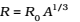### Basic Properties of Neutron

• mn = 1.00866 u = 1.6749 × 10-27 kg
• A free neutron, unlike a free proton, is unstable
• It decays into a proton, an electron and an antineutrino (another elementary particle), and has a mean life of about 1000s. It is, however, stable inside the nucleus.

### Composition of Nucleus

The composition of a nucleus can now be described using the following terms and symbols:

Z – Atomic number = number of protons

N – Neutron number = number of neutrons

A – Mass number = Z + N = total number of protons and neutrons

One also uses the term nucleon for a proton or a neutron. Thus the number of nucleons in an atom is its mass number A. Nuclear species or nuclides are shown by the notation where X is the chemical symbol of the species. For example, the nucleus of gold is denoted by. It contains 197 nucleons, of which 79 are protons and the rest118 are neutrons.

### Size of the Nucleus

It has been found that a nucleus of mass number A has a radiuswhere R0 = 1.2 × 10-15 m.

This means the volume of the nucleus, which is proportional to R3 is proportional to A. Thus the density of nucleus is a constant, independent of A, for all nuclei. Different nuclei are likes drop of liquid of constant density.

The density of nuclear matter is approximately 2.3 × 1017 kg m-3. This density is very large compared to ordinary matter, say water, which is 103 kg m-3.

### Isotopes:

The nuclei of isotopes of a given element contain the same number of protons, but differ from each other in their number of neutrons.

### Isobars:

All nuclides with same mass number are called isobars.

### Isotones:

Nuclei of different atom containing same number of neutrons are called isotones.